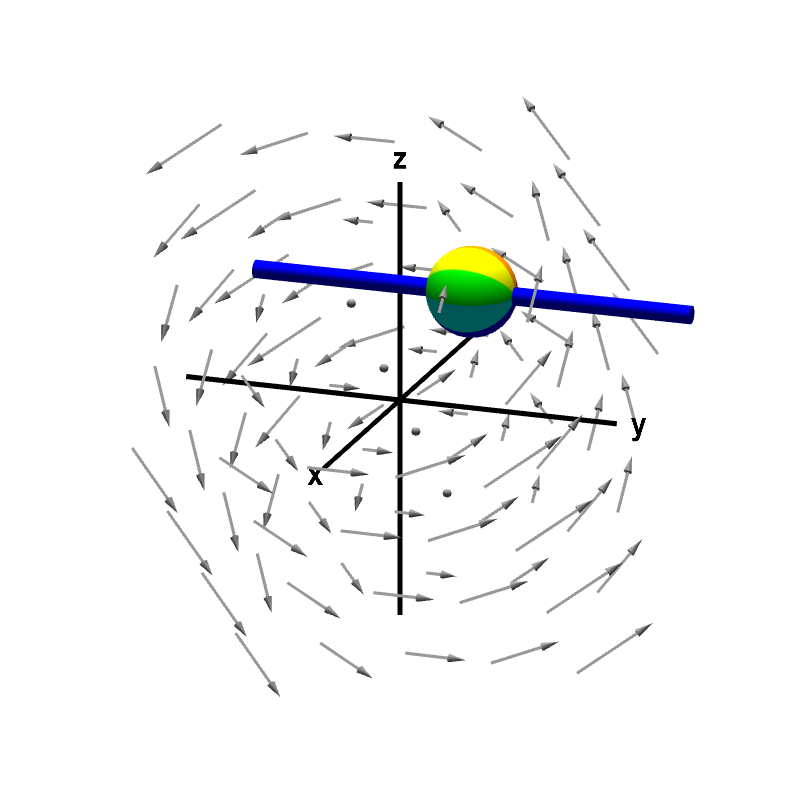# Math Insight

### Applet: A nonrotating sphere on a rod shows no y-component of curlThe rod constrains the rotation of the sphere to point (using the right hand rule) in either the positive or negative $y$ direction. The speed of this rotation corresponds to the $y$-component of the curl. In this case, the sphere does not rotate, indicating the $y$-component of the curl is zero.

This vector field is $\dlvf(x,y,z)= (-y,x-z,y).$ Its curl is \begin{align*} \curl \dlvf(x,y,z) &= \left(\pdiff{}{y}y-\pdiff{}{z}(x-z), \pdiff{}{z}(-y)- \pdiff{}{x}y, \pdiff{}{x}(x-z) - \pdiff{}{y}(-y) \right)\\ &=(2,0,2). \end{align*} In particular, the $y$-component corresponding to the sphere's (lack of) rotation is 0. Since the $y$-component of the curl is zero everywhere, the sphere does not spin no matter where you move it.